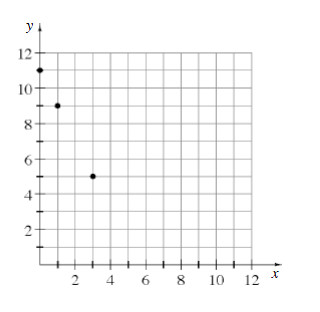### Home > MC1 > Chapter 7 > Lesson 7.3.1 > Problem7-109

7-109.

Look at the graph below.1. Write the coordinates $\left(x, y\right)$ of the three points shown on the graph.

Refer to the Lesson 4.1.2 Math Notes box for how to graph points on an $xy$ - coordinate grid.

2. Write the coordinates $\left(x, y\right)$ of two other points that would be in line with the three points shown.

Draw a line connecting these three points. What other points are on this line?

One point on the line is $\left(5, 1\right)$. Can you find at least one more point on the line?